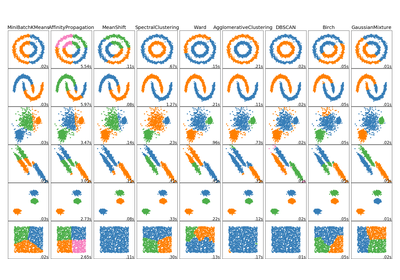# `sklearn.cluster`.SpectralClustering¶

class `sklearn.cluster.``SpectralClustering`(n_clusters=8, eigen_solver=None, random_state=None, n_init=10, gamma=1.0, affinity=’rbf’, n_neighbors=10, eigen_tol=0.0, assign_labels=’kmeans’, degree=3, coef0=1, kernel_params=None, n_jobs=1)[source]

Apply clustering to a projection to the normalized laplacian.

In practice Spectral Clustering is very useful when the structure of the individual clusters is highly non-convex or more generally when a measure of the center and spread of the cluster is not a suitable description of the complete cluster. For instance when clusters are nested circles on the 2D plan.

If affinity is the adjacency matrix of a graph, this method can be used to find normalized graph cuts.

When calling `fit`, an affinity matrix is constructed using either kernel function such the Gaussian (aka RBF) kernel of the euclidean distanced `d(X, X)`:

```np.exp(-gamma * d(X,X) ** 2)
```

or a k-nearest neighbors connectivity matrix.

Alternatively, using `precomputed`, a user-provided affinity matrix can be used.

Read more in the User Guide.

Parameters: n_clusters : integer, optional The dimension of the projection subspace. eigen_solver : {None, ‘arpack’, ‘lobpcg’, or ‘amg’} The eigenvalue decomposition strategy to use. AMG requires pyamg to be installed. It can be faster on very large, sparse problems, but may also lead to instabilities random_state : int, RandomState instance or None, optional, default: None A pseudo random number generator used for the initialization of the lobpcg eigen vectors decomposition when eigen_solver == ‘amg’ and by the K-Means initialization. If int, random_state is the seed used by the random number generator; If RandomState instance, random_state is the random number generator; If None, the random number generator is the RandomState instance used by np.random. n_init : int, optional, default: 10 Number of time the k-means algorithm will be run with different centroid seeds. The final results will be the best output of n_init consecutive runs in terms of inertia. gamma : float, default=1.0 Kernel coefficient for rbf, poly, sigmoid, laplacian and chi2 kernels. Ignored for `affinity='nearest_neighbors'`. affinity : string, array-like or callable, default ‘rbf’ If a string, this may be one of ‘nearest_neighbors’, ‘precomputed’, ‘rbf’ or one of the kernels supported by sklearn.metrics.pairwise_kernels. Only kernels that produce similarity scores (non-negative values that increase with similarity) should be used. This property is not checked by the clustering algorithm. n_neighbors : integer Number of neighbors to use when constructing the affinity matrix using the nearest neighbors method. Ignored for `affinity='rbf'`. eigen_tol : float, optional, default: 0.0 Stopping criterion for eigendecomposition of the Laplacian matrix when using arpack eigen_solver. assign_labels : {‘kmeans’, ‘discretize’}, default: ‘kmeans’ The strategy to use to assign labels in the embedding space. There are two ways to assign labels after the laplacian embedding. k-means can be applied and is a popular choice. But it can also be sensitive to initialization. Discretization is another approach which is less sensitive to random initialization. degree : float, default=3 Degree of the polynomial kernel. Ignored by other kernels. coef0 : float, default=1 Zero coefficient for polynomial and sigmoid kernels. Ignored by other kernels. kernel_params : dictionary of string to any, optional Parameters (keyword arguments) and values for kernel passed as callable object. Ignored by other kernels. n_jobs : int, optional (default = 1) The number of parallel jobs to run. If `-1`, then the number of jobs is set to the number of CPU cores. affinity_matrix_ : array-like, shape (n_samples, n_samples) Affinity matrix used for clustering. Available only if after calling `fit`. labels_ : : Labels of each point

Notes

If you have an affinity matrix, such as a distance matrix, for which 0 means identical elements, and high values means very dissimilar elements, it can be transformed in a similarity matrix that is well suited for the algorithm by applying the Gaussian (RBF, heat) kernel:

```np.exp(- dist_matrix ** 2 / (2. * delta ** 2))
```

Where `delta` is a free parameter representing the width of the Gaussian kernel.

Another alternative is to take a symmetric version of the k nearest neighbors connectivity matrix of the points.

If the pyamg package is installed, it is used: this greatly speeds up computation.

References

Methods

 `fit`(X[, y]) Creates an affinity matrix for X using the selected affinity, then applies spectral clustering to this affinity matrix. `fit_predict`(X[, y]) Performs clustering on X and returns cluster labels. `get_params`([deep]) Get parameters for this estimator. `set_params`(**params) Set the parameters of this estimator.
`__init__`(n_clusters=8, eigen_solver=None, random_state=None, n_init=10, gamma=1.0, affinity=’rbf’, n_neighbors=10, eigen_tol=0.0, assign_labels=’kmeans’, degree=3, coef0=1, kernel_params=None, n_jobs=1)[source]
`fit`(X, y=None)[source]

Creates an affinity matrix for X using the selected affinity, then applies spectral clustering to this affinity matrix.

Parameters: X : array-like or sparse matrix, shape (n_samples, n_features) OR, if affinity==`precomputed`, a precomputed affinity matrix of shape (n_samples, n_samples) y : Ignored
`fit_predict`(X, y=None)[source]

Performs clustering on X and returns cluster labels.

Parameters: X : ndarray, shape (n_samples, n_features) Input data. y : ndarray, shape (n_samples,) cluster labels
`get_params`(deep=True)[source]

Get parameters for this estimator.

Parameters: deep : boolean, optional If True, will return the parameters for this estimator and contained subobjects that are estimators. params : mapping of string to any Parameter names mapped to their values.
`set_params`(**params)[source]

Set the parameters of this estimator.

The method works on simple estimators as well as on nested objects (such as pipelines). The latter have parameters of the form `<component>__<parameter>` so that it’s possible to update each component of a nested object.

Returns: self :

## Examples using `sklearn.cluster.SpectralClustering`¶Comparing different clustering algorithms on toy datasets